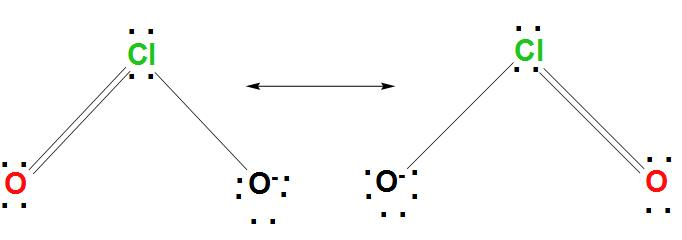# How can I draw the Lewis structure for $Cl{{O}^{2-}}$ ?Verified
176.1k+ views
Hint: As we know that Lewis structures are the diagrams that show the bonding between atoms of a molecule and the lone pairs of electrons that can exist in the molecule. It is found that these are also called electron dot structures. It basically adds up two lines between the atoms that shows shared pairs.

Complete Solution :
- In order to draw the Lewis structure for $Cl{{O}^{2-}}$, we can find the procedure here.
- As we know that for $Cl{{O}^{2-}}$, Cl is found to be the less electronegative atom, hence Cl is the central atom. The skeletal structure of Cl is found to be represented as:
O-Cl-O
- It is found that every atom has a formal charge. We can reduce the number of formal charges by moving a lone pair of electrons from oxygen to form a Cl=O bond.
- And we get two new structures in which one of the oxygen atoms has a formal charge equal to -1, and the other two atoms have a formal charge equal to zero.
- We can draw the Lewis structure for $Cl{{O}^{2-}}$as:Note: - It is found that octet rule exists because the atoms of most of the elements become more stable by attaining the electronic configuration of an element. As we know that in octet rule atoms prefer to have eight electrons in the valence shell.
- Lewis structure mainly describes the structure of molecules with the help of symbols for the chemical species and also dot symbols.